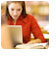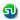### Linear Equation, Simultaneous equation. TANCET Quant Preparation. Ascent TANCET MBA Classes

 Home TANCET Classes GMAT Coaching Online GMAT Course CAT Classes TANCET Study Material
 TANCET '18 Classroom ProgramWeekend and weekday classes for TANCET MBA @ Chennai. At Nungambakkam and Velachery.Other Courses TANCET Correspondence Course CAT Classes & Courses GMAT Classes Chennai Online GMAT Course GRE Classes Chennai CBSE Math Online Tuition SAT Classes Other Links Ascent TANCET Toppers Testimonials CAT, TANCET Questions Careers @ Ascent Contact Us +91 44 4500 8484 +91 96000 48484 ascent@ascenteducation.com Postal Address Facebook / Twitter / Blog / Videos
You are here: Home  »  CAT, XAT, TANCET Prep Questions »  Linear Equations »   Question 9

# Math Problems : Simultaneous Equations

## Question

Rajesh is 10 years younger to Baskar. 10 years back, Rajesh's age was two-thirds that of Baskar's. How old is Baskar now?
1. 30
2. 40
3. 20
4. 16
5. 28

Choice (2). 40

Let the present age of Baskar be 'b' and that of Rajesh be 'r'.
So, r = b - 10 .... eqn (1).

10 years back Rajesh was (r - 10) years old. 10 years back Baskar was (b - 10) years old.

The question states that 10 years back Rajesh was two thirds as old as Baskar was.
i.e., (r - 10) = (2/3)*(b - 10) .... eqn (2).

Cross multiplying, we get 3(r - 10) = 2(b - 10)
or 3r - 30 = 2b - 20 .... eqn (2)

From eqn (1) we can substitute r as (b - 10) in eqn (2)
So, 3(b - 10) - 30 = 2b - 20
or 3b - 30 - 30 = 2b - 20
or b = 40.

The present age of Baskar is 40 years.

## More Questions on Linear, Quadratic and Cubic Equations - Algebra

 5. 6. 7.

## CAT, XAT, TANCET Practice Questions and Answers : Listed Topicwise

 Number Theory Permutation Combination Probability Inequalities Geometry Mensuration Trigonometry Coordinate Geometry Percentages Profit Loss Ratio Proportion Mixtures Alligation Speed Time Distance Pipes Cisterns Interest Races Average, Mean AP, GP, HP Set Theory Clocks Calendars Algebra Function English Grammar General Awareness Data sufficiency TANCET Papers XAT PapersAdd to del.icio.usStumble Itdigg this